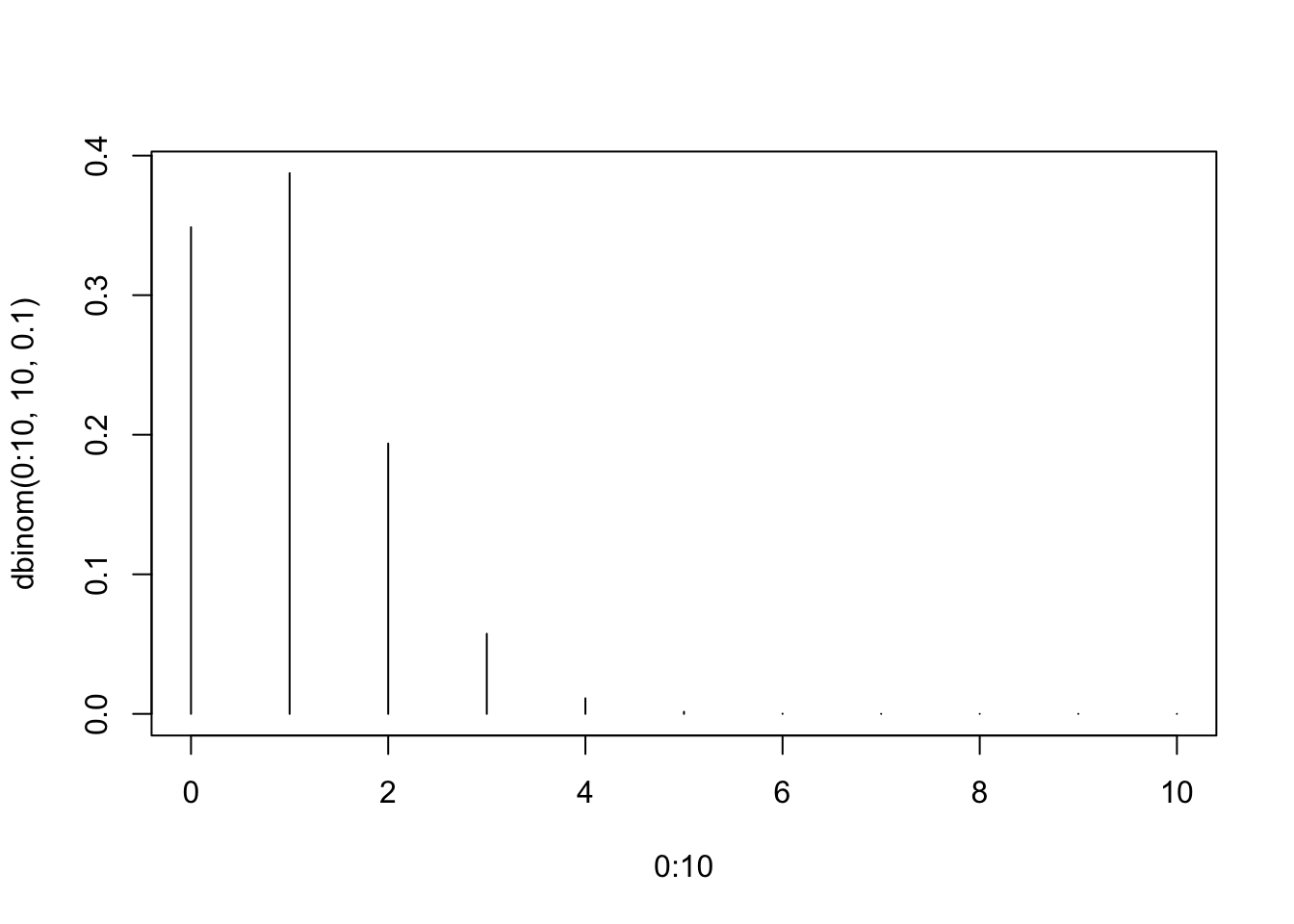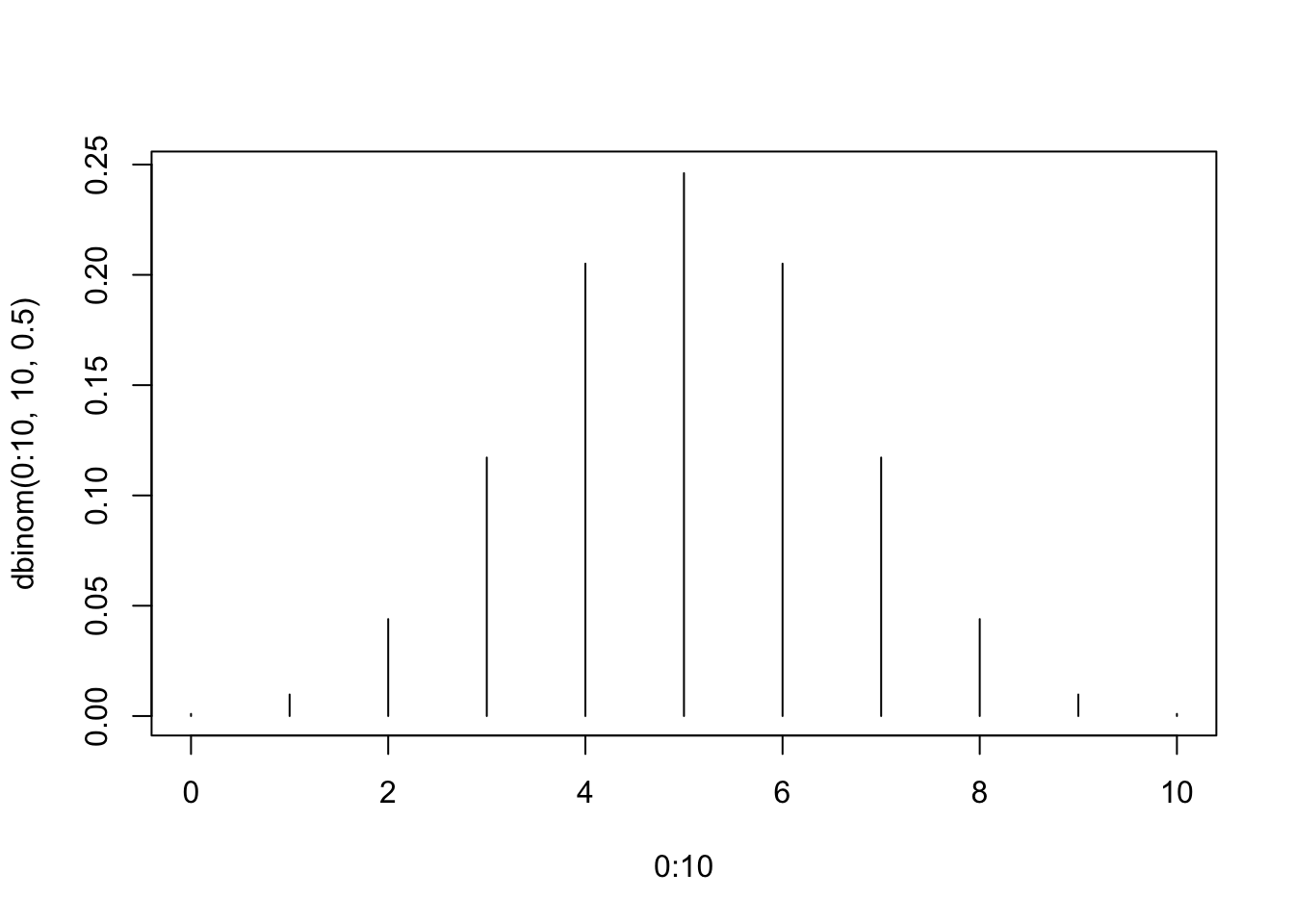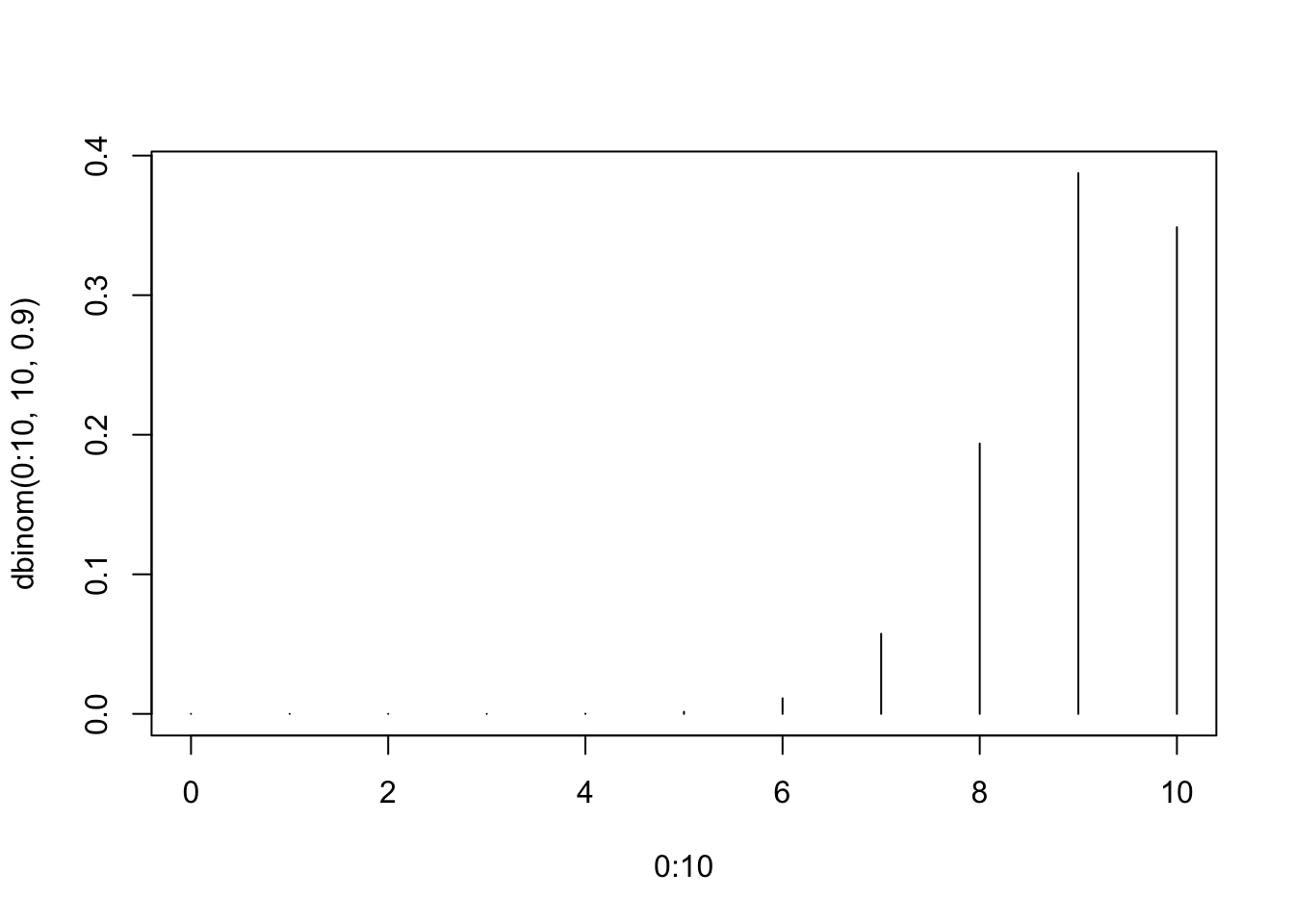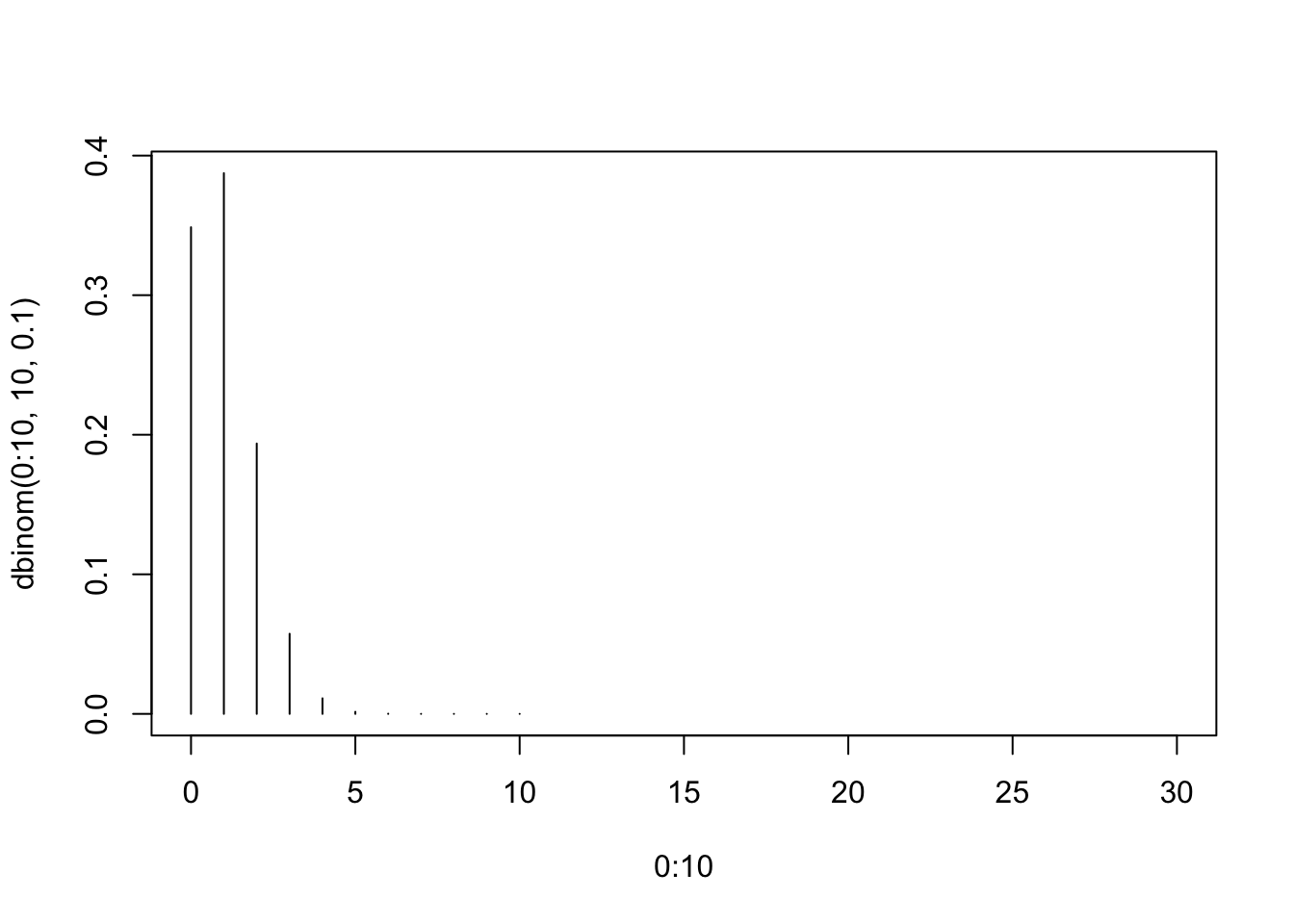### Computing binomial probabilities

Let $$X \sim bin(n = 10, \pi = 0.1)$$. If you are interested in computing $$P(X = x), \forall x = 0, \ldots, k$$, you can exploit the dbinom function.

The first argument of dbinom is the value of $$x$$ in which you are interested, the second argument is the value of $$n$$ and the third argument is the value of $$\pi$$. Therefore, $$P(X = 0)$$ is:

dbinom(x = 0, size = 10, prob = 0.1)
##  0.3486784

As with all R functions, you can exploit the “calling by position” syntax, and obtain the same result through the following call:

dbinom(x = 0, size = 10, prob = 0.1)
##  0.3486784

The dbinom function is vectorized. You can obtain the whole probability distribution specifying as first argument the whole set of admissible values for $$x$$:

dbinom(0:10, 10, 0.1)
##   0.3486784401 0.3874204890 0.1937102445 0.0573956280 0.0111602610
##   0.0014880348 0.0001377810 0.0000087480 0.0000003645 0.0000000090
##  0.0000000001

Finally you can easily plot the binomial distribution as follows:

plot(0:10, dbinom(0:10, 10, 0.1), type="h")### The shape of the binomial distribution

The binomial distribution is perfectly symmetric when $$\pi = 0.5$$, for any value of $$n$$. Here is an example:

plot(0:10, dbinom(0:10, 10, 0.5), type="h")The binomail distribution is left-skewed when the $$\pi < 0.5$$ while is right-skewed when $$\pi > 0.5$$ (Why?).

Follows two examples for $$\pi = 0.1$$ and $$\pi = 0.9$$, respectively:

plot(0:10, dbinom(0:10, 10, 0.1), type="h")plot(0:10, dbinom(0:10, 10, 0.9), type="h")Finally, when $$n$$ increases, the binomial distribution tends to be symmetric (effect of the central limit theorem, version of the De Moivre-Laplace) independently of the value of $$\pi$$. About that, see the following plots depicting the binomial law for $$\pi = 0.1$$ and for $$n = 10, 30, 100$$:

plot(0:10, dbinom(0:10, 10, 0.1), type="h", xlim = c(0, 30))plot(0:30, dbinom(0:30, 30, 0.1), type="h", xlim = c(0, 30))